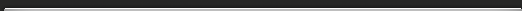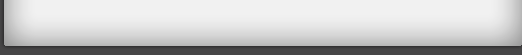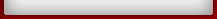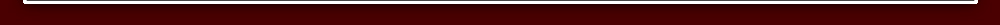Pregnancy Calculator

# Pregnancy Calculator

Use the Pregnancy Calculator to:

• Determine the due date
• Calculate the likely conception date
• Calculate the end of the first and second trimesters
• Find out for how many weeks have you been pregnant
• Calculate how many weeks you will be pregnant on a given date
• Calculate on which date you will be pregnant the specified number of weeks, and
• Determine the likely conception date when you know the birthday or due date.

## Pregnancy Calculator

When was the first day of your last menstrual period?

Number of days in your menstrual cycle
Likely conception date:
End of first trimester (12 weeks):
End of second trimester (27 weeks):
Your due date is (40 weeks):
Number of weeks you have been pregnant for:
How many weeks will you be pregnant on a given date?

Number of weeks you will be pregnant for:
On which date will you be pregnant for the given number of weeks?
The number of weeks:
Calculated date:
Given the birthday or due date, calculate likely conception date

Calculated conception date:
Copyright © 2008 Muzamal . All rights reserved.

## How to use the Pregnancy Calculator

Enter the date of your last menstrual period and the number of days in your menstrual cycle into the pregnancy calculator, and click on the Calculate button. Other calculations are optional - use them when needed.

On average, there are 28 days in a menstrual cycle, but it can last from as little as 22 to as many as 44 days. The Pregnancy Calculator takes your menstrual cycle into account when calculating the due date and the conception date.

Also, the Pregnancy Calculator needs the number of days in your menstrual cycle when calculating the likely conception date from the given birthday or the due date.

Join My Forum

Set as your home pageWeb StatusAdvertisementBecome FanLink Partners=> Do you also want a homepage for free? Then click here! <=